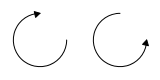# OEDrawCurvedArrow¶

void OEDrawCurvedArrow(OEImageBase& image, const OE2DPoint& center,
double bgnAngle, double endAngle, double radius,
bool clockwise, const OEPen& pen);


Draws a curved arrow. See example in Figure: Example of drawing curved arrows.Example of drawing curved arrows

image

The image in which the arrow is drawn.

center

The center of the arc.

bgnAngle, endAngle

The two endpoints of the arc (in degrees). Both angles are in degrees and their values have to be in a range of $$[0.0^{\circ}, 360.0^{\circ}]$$. Angles are interpreted such that $$0.0^{\circ}$$ and $$360.0^{\circ}$$ degrees are at the 12 o’clock position, $$90.0^{\circ}$$ degrees corresponds to 3 o’clock, etc.